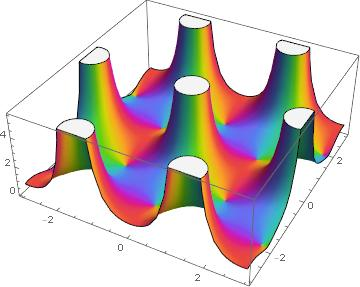# Not even closeVery often what cannot be done exactly can be done approximately. For example, most integrals cannot be computed in closed form, but they can be calculated numerically as closely as you’d like. But sometimes things are impossible, and you can’t even come close.

## An impossible assignment

When I was in college, I had a friend who had a job in an engineering lab. The director of his lab had asked him to find an analytic analog to a smoothed indicator function. (I’ll say in more detail what that means shortly.) He mentioned the problem to me, and I told him there’s a theorem that says his assignment is impossible .

My friend was not deterred by my answer, confident that as an engineer he could find a practical way to do what mathematicians say can’t be done. That’s often a useful attitude, though not in this instance. Looking back, I can see how I could have given him a more useful reply than simply saying his task couldn’t be done; I could have explained why it cannot even be done approximately.

## No exact plateaus

The indicator function of an interval is a function that takes on the value 1 on the interval and 0 everywhere else. This is a discontinuous function, but it can be approximated by a smooth function. Given a little transition zone on either end, you can have the function be zero on one side, one on the other, and ramp up smoothly in between. You can do this with infinitely differentiable functions, so why can’t you do something similar with analytic functions?

An analytic function can be expressed as a power series, and a power series is determined by its values in a small neighborhood of where it is centered. If you look at a patch where the function is zero, all its derivatives are zero, and so all the series coefficients are zero, and the function is zero. So the function’s value is zero everywhere. An analytic function can’t have a plateau without being flat everywhere.

## No approximate plateaus

But can an analytic function have an approximate plateau? Can you construct an analytic function that is nearly 1 on some region, say a disk, and nearly 0 outside of some thin boundary around the disk ? In more picturesque language, can you construct an analytic function whose absolute value looks like Devil’s Tower in the photo above?

The barrier to creating something like Devil’s Tower is the maximum modulus principle. It says that the absolute value of an analytic function cannot have an interior maximum; the maximum always occurs on the boundary.

Suppose you’re trying to construct a function f(z) such that |f(z)| is a approximately zero within a radius 1 of the origin and approximately 0 outside a disk of radius 2. The first part is possible but the second part is not. The function f(z) cannot be perfectly flat on top without being constant everywhere (Liouville’s theorem) and the maximum of |f(z)| over the unit disk must occur somewhere on the rim of the disk (maximum modulus principle). However, it could be that f doesn’t vary much on the disk, and so there’s not much difference between its maximum and minimum over the disk.

Now consider the disk of radius 2. Somewhere on the rim, the circle of radius 2, |f(z)| must be larger than it was on the unit disk, or else |f(z)| would have an interior maximum, which the maximum modulus principle says cannot happen. |f(z)| might be small along parts of the circle of radius 2, but somewhere on that circle it is approximate 1 or larger.

## Fake plateaus

Sometimes you will see plots of analytic functions that do look flat on top, but that’s because singularities have been chopped off. Here’s such a plot from a blog post in a while back, a plot of Weierstrass’ elliptic function. The white plateaus are artifacts of cutting infinite values to fit in a finite box.## Related posts

 I wondered for a second how my friend’s supervisor couldn’t know this, then I realized it was probably a setup. His supervisor had given him an impossible task so that he’d struggle with it and learn why it was impossible.

 You could construct an analytic function that approximates a plateau along a one-dimensional slice, say along the real axis, but that approximation cannot be good in all directions.

## 2 thoughts on “Not even close”

1. Nathan Hannon

Is it clear from the context that “analytic” means complex? (I’ve seen the term “holomorphic” used more often in reference to complex functions.) If he was talking about real analytic functions, the picture is quite different. You still can’t get an exact indicator function, but you can get close with, say, Fourier series or Weierstrass polynomials.

2. Tim Stoev

what if you put that in some weird space with variable metrics?